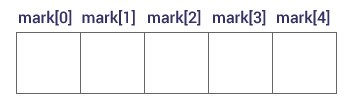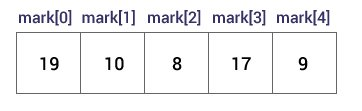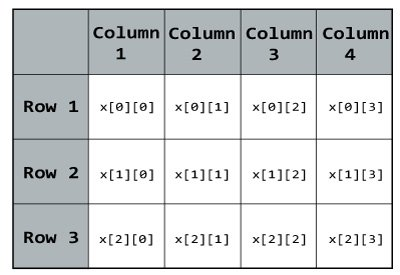# C Programming Arrays

In C language, arrays are referred to as structured data types. An array is a collection of data that holds fixed number of values of same type.

OR

An array is defined as finite ordered collection of homogenous data, stored in contiguous memory locations.

Here the words,

1. finite means data range must be defined.
2. ordered means data must be stored in continuous memory addresses.
3. homogenous means data must be of similar data type.

For example: if you want to store marks of 100 students, you can create an array for it.

`float marks;`

The size and type of arrays cannot be changed after its declaration.

Arrays are of two types:

1. One-dimensional arrays
2. Multidimensional arrays

### How to declare an array in C?

`data_type array_name[array_size];`

For example,

`float mark;`

Here, we declared an array, `mark`, of floating-point type and size 5. Meaning, it can hold 5 floating-point values.

### Elements of an Array and How to access them?

You can access elements of an array by indices.

Suppose you declared an array `mark` as above. The first element is `mark`, second element is `mark` and so on.#### Few key notes:

1. Arrays have 0 as the first index not 1. In this example, `mark`
2. If the size of an array is `n`, to access the last element, `(n-1)` index is used. In this example, `mark`
3. Suppose the starting address of `mark` is 2120d. Then, the next address, `a`, will be 2124d, address of `a` will be 2128d and so on. It's because the size of a float is 4 bytes.

### How to initialize an array in C programming?

It's possible to initialize an array during declaration. For example,

`int mark = {19, 10, 8, 17, 9};`

Another method to initialize array during declaration:

`int mark[] = {19, 10, 8, 17, 9};`Here,

```mark is equal to 19
mark is equal to 10
mark is equal to 8
mark is equal to 17
mark is equal to 9```

### How to insert and print array elements?

``````int mark = {19, 10, 8, 17, 9}

// insert different value to third element
mark = 9;

// take input from the user and insert in third element
scanf("%d", &mark);

// take input from the user and insert in (i+1)th element
scanf("%d", &mark[i]);

// print first element of an array
printf("%d", mark);

// print ith element of an array
printf("%d", mark[i-1]);``````

MIND IT !

After an array is declared it must be initialized. Otherwise, it will contain garbage value(any random value). An array can be initialized at either compile time or at runtime.

Compile time Array initialization

Compile time initialization of array elements is same as ordinary variable initialization. The general form of initialization of array is,

``````type array-name[size]= {list of values};
int marks = {19, 10, 8, 17}  // integer array initialization
float area = {23.4, 6.8, 5.5} // float array initialization
int marks = {19, 10, 8, 17, 9} // Compile time error

``````

One important thing to remember is that when you will give more initializer(array elements) than declared array size than the compiler will give an error.

``````#include<stdio.h>
#include<conio.h>
void main()
{
int i;
int arr[]={2,3,4};    //Compile time array initialization
for(i=0 ; i<3 ; i++) {
printf("%d\t",arr[i]);
}
getch();
}
```
```

Output

`2 3 4`

Runtime Array initialization

An array can also be initialized at runtime using `scanf()` function. This approach is usually used for initializing large array, or to initialize array with user specified values. Example,

``````#include<stdio.h>
#include<conio.h>
void main()
{
int arr;
int i, j;
printf("Enter array element");
for(i=0;i<4;i++)
{
scanf("%d",&arr[i]);    //Run time array initialization
}
for(j=0;j<4;j++)
{
printf("%d\n",arr[j]);
}
getch();
}
```
```

### Example: C Arrays

``````// Program to find the average of n (n < 10) numbers using arrays

#include <stdio.h>
int main()
{
int marks, i, n, sum = 0, average;
printf("Enter n: ");
scanf("%d", &n);
for(i=0; i<n; ++i)
{
printf("Enter number%d: ",i+1);
scanf("%d", &marks[i]);
sum += marks[i];
}
average = sum/n;

printf("Average marks = %d", average);

return 0;
}``````
Output

```Enter n: 5
Enter number1: 45
Enter number2: 35
Enter number3: 38
Enter number4: 31
Enter number5: 49
Average = 39```

### Important thing to remember when working with C arrays

Suppose you declared an array of 10 elements. Let's say,

`int testArray;`

You can use the array members from `testArray` to `testArray`.

If you try to access array elements outside of its bound, let's say `testArray`, the compiler may not show any error. However, this may cause unexpected output (undefined behavior).

C Programming Multidimensional Arrays

In C programming, you can create an array of arrays known as multidimensional array. For example,

Here, `x` is a two-dimensional (2d) array. The array can hold 12 elements. You can think the array as table with 3 row and each row has 4 column.Similarly, you can declare a three-dimensional (3d) array. For example,

`float y;`

Here,The array `y` can hold 24 elements.

You can think this example as: Each 2 elements have 4 elements, which makes 8 elements and each 8 elements can have 3 elements. Hence, the total number of elements is 24.

### How to initialize a multidimensional array?

There is more than one way to initialize a multidimensional array.

### Initialization of a two dimensional array.

```// Different ways to initialize two dimensional array

int c = {{1, 3, 0}, {-1, 5, 9}};

int c[] = {{1, 3, 0}, {-1, 5, 9}};

int c = {1, 3, 0, -1, 5, 9};```
Above code are three different ways to initialize a two dimensional arrays.

### Initialization of a three dimensional array.

You can initialize a three dimensional array in a similar way like a two dimensional array. Here's an example,

```int test = {
{ {3, 4, 2, 3}, {0, -3, 9, 11}, {23, 12, 23, 2} },
{ {13, 4, 56, 3}, {5, 9, 3, 5}, {3, 1, 4, 9} }
};```

Example #1: Two Dimensional Array to store and display values

``````// C program to store temperature of two cities for a week and display it.
#include <stdio.h>

const int CITY = 2;
const int WEEK = 7;

int main()
{
int temperature[CITY][WEEK];
for (int i = 0; i < CITY; ++i) {
for(int j = 0; j < WEEK; ++j) {
printf("City %d, Day %d: ", i+1, j+1);
scanf("%d", &temperature[i][j]);
}
}

printf("\nDisplaying values: \n\n");
for (int i = 0; i < CITY; ++i) {
for(int j = 0; j < WEEK; ++j)
{
printf("City %d, Day %d = %d\n", i+1, j+1, temperature[i][j]);
}
}
return 0;
}``````

Output

```City 1, Day 1: 33
City 1, Day 2: 34
City 1, Day 3: 35
City 1, Day 4: 33
City 1, Day 5: 32
City 1, Day 6: 31
City 1, Day 7: 30
City 2, Day 1: 23
City 2, Day 2: 22
City 2, Day 3: 21
City 2, Day 4: 24
City 2, Day 5: 22
City 2, Day 6: 25
City 2, Day 7: 26

Displaying values:

City 1, Day 1 = 33
City 1, Day 2 = 34
City 1, Day 3 = 35
City 1, Day 4 = 33
City 1, Day 5 = 32
City 1, Day 6 = 31
City 1, Day 7 = 30
City 2, Day 1 = 23
City 2, Day 2 = 22
City 2, Day 3 = 21
City 2, Day 4 = 24
City 2, Day 5 = 22
City 2, Day 6 = 25
City 2, Day 7 = 26```

### Example #2: Sum of two matrices using Two dimensional arrays

C program to find the sum of two matrices of order 2*2 using multidimensional arrays.

``````#include <stdio.h>
int main()
{
float a, b, c;
int i, j;

// Taking input using nested for loop
printf("Enter elements of 1st matrix\n");
for(i=0; i<2; ++i)
for(j=0; j<2; ++j)
{
printf("Enter a%d%d: ", i+1, j+1);
scanf("%f", &a[i][j]);
}

// Taking input using nested for loop
printf("Enter elements of 2nd matrix\n");
for(i=0; i<2; ++i)
for(j=0; j<2; ++j)
{
printf("Enter b%d%d: ", i+1, j+1);
scanf("%f", &b[i][j]);
}

// adding corresponding elements of two arrays
for(i=0; i<2; ++i)
for(j=0; j<2; ++j)
{
c[i][j] = a[i][j] + b[i][j];
}

// Displaying the sum
printf("\nSum Of Matrix:");

for(i=0; i<2; ++i)
for(j=0; j<2; ++j)
{
printf("%.1f\t", c[i][j]);

if(j==1)
printf("\n");
}
return 0;
}``````

Output

```Enter elements of 1st matrix
Enter a11: 2;
Enter a12: 0.5;
Enter a21: -1.1;
Enter a22: 2;
Enter elements of 2nd matrix
Enter b11: 0.2;
Enter b12: 0;
Enter b21: 0.23;
Enter b22: 23;

Sum Of Matrix:
2.2     0.5
-0.9    25.0```
LearnFrenzy provides you lots of fully solved "C Programming Array" Questions and Answers with explanation.